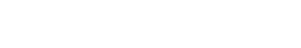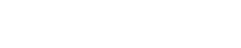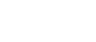# Stephen Senn: “Delta Force: To what extent is clinical relevance relevant?” (Guest Post)

Stephen Senn
Competence Center for Methodology and Statistics (CCMS),
Luxembourg

Delta Force
To what extent is clinical relevance relevant?

Inspiration
This note has been inspired by a Twitter exchange with respected scientist and famous blogger  David Colquhoun. He queried whether a treatment that had 2/3 of an effect that would be described as clinically relevant could be useful. I was surprised at the question, since I would regard it as being pretty obvious that it could but, on reflection, I realise that things that may seem obvious to some who have worked in drug development may not be obvious to others, and if they are not obvious to others are either in need of a defence or wrong. I don’t think I am wrong and this note is to explain my thinking on the subject.

Conventional power or sample size calculations
As is happens, I don’t particularly like conventional power calculations but I think they are, nonetheless, a good place to start. To carry out such a calculation a statistician needs the following ingredients

1. A definition of a rational design (the smallest design that is feasible but would retain the essential characteristics of the design chosen).
2. An agreed outcome measure.
3. A proposed analysis.
4. A measure of variability for the rational design. (This might, for example, be the between-patient variance σ2 for a parallel group design.)
5. An agreed type I error rate, α.
6. An agreed power, 1-β.
7. A clinically relevant difference, δ. (To be discussed.)
8. The size of the experiment, n, (in terms of multiples of the rational design).

In treatments of this subject points 1-3 are frequently glossed over as already being known and given, although in my experience, any serious work on trial design involves the statistician in a lot of work investigating and discussing these issues. In consequence, in conventional discussions, attention is placed on points 4-8. Typically, it is assumed that 4-7 are given and 8, the size of the experiment, is calculated as a consequence. More rarely, 4, 5, 7 and 8 are given and 6, the power, is calculated from the other 4. An obvious weakness of this system is that there is no formal mention of cost whether in money, lost opportunities or patient time and suffering.

An example
A parallel group trial is planned in asthma with 3 months follow up. The agreed outcome measure is forced expiratory volume in one second (FEV1) at the end of the trial. The between-patient standard deviation is 450ml and the clinically relevant difference is 200ml. A type I error rate of 5% is chosen and the test will be two-sided. A power of 80% is targeted.

An approximate formula that may be used isHere the second term on the right hand side reflects what I call decision precision, with zα/2, zβ  as the relevant percentage points of the standard Normal. If you lower the type I error rate or increase the power, decision precision will increase. The first term on the right hand side is the variance for a rational design (consisting of one patient on each arm) expressed as a ratio to the square of a clinically relevant difference. It is a noise-to-signal ratio.

Substituting we haveThus we need an 80-fold replication of the rational design, which is to say, 80 patients on each arm.

What is delta?
I now list different points of view regarding this.

1.     It is the difference we would like to observe
This point of view is occasionally propounded but it is incompatible with the formula used. To see this consider a re-arrangement of equation (1) asThe numerator on the left hand side is the clinically relevant difference and the denominator is the standard error. Now if the observed difference, d,  is the same as the clinically relevant difference the we can replace δ by d in (2) but that would imply that the ratio of observed value to statistic would be (in our example) 2.8. This does not correspond to a P-value of 0.05, which our calculation was supposed to deliver us with 80% probability if the clinically relevant difference obtained but to a P-value of 0.006, or just over 1/10 of what our power calculation would accept as constituting proof of efficacy.

To put it another way if δ is the value we would like to observe and if the treatment does, indeed, have a value of δ then we have only half a chance, not an 80% chance, that the trial will deliver to us a value as big as this.

2.     It is the difference we would like to ‘prove’ obtains
This view is hopeless. It requires that the lower confidence interval should be greater than δ. If this is what is needed, the power calculation is completely irrelevant.

3.     It is the difference we believe obtains
This is another wrong-headed notion. Since, the smaller the value of δ the larger the sample size, it would have the curious side effect that given a number of drug-development candidates we would spend most money on those we considered least promising. There are some semi-Bayesian versions of this in which a probability distribution for δ would be substituted for a single value. Most medical statisticians would reject this as being a pointless elaboration of a point of view that is wrong in the first place. If you reject the notion that δ is your best guess as to what the treatment effect is there is no need to elaborate this rejected position by giving δ a probability distribution.

Note, I am not rejecting the idea of Bayesian sample size calculations. A fully decision-analytic approach might be interesting.  I am rejecting what is a Bayesian-frequentist chimera.

4.     It is the difference you would not like to miss
This is the interpretation I favour. The idea is that we control two (conditional) errors in the process. The first is α, the probability of claiming that a treatment is effective when it is, in fact, no better than placebo. The second is the error of failing to develop a (very) interesting treatment further. If a trial in drug development is not ‘successful’, there is a chance that the whole development programme will be cancelled. It is the conditional probability of cancelling an interesting project that we seek to control.

Note that the FDA will usually requires that two phase III trials are ‘significant’ and significance requires that the observed effect is at least equal toIn our example this would give us 1.96/2.8=0.7δ, or a little over two thirds of δ for at least two trials for any drug that obtained registration. In practice, the observed average of the two would have an effect somewhat in excess of 0.7δ. Of course, we would be naïve to believe that all drugs that get accepted have this effect (regression to the mean is ever- present) but nevertheless it provides some reassurance.

Lessons
In other words, if you are going to do a power calculation and you are going to target some sort of value like 80% power, you need to set δ at a value that is higher than that you would be happy to find. Statisticians like me think of δ as the difference we would not like to miss and we call this the clinically relevant difference.

Does this mean that an effect that is 2/3 of the clinically relevant difference is worth having? Not necessarily. That depends on what your understanding of the phrase is. It should be noted, however, that when it is crucial to establish that no important difference between treatments exists, as in a non-inferiority study, then another sort of difference is commonly used. This is referred to as the clinically irrelevant difference. Such differences are quite commonly no more than 1/3 of the sort of difference a drug will have shown historically to placebo and hence much smaller than the difference you would not like to miss.

Another lesson, however, is this. In this area, as in others in the analysis of clinical data, dichotomisation is a bad habit. There are no hard and fast boundaries. Relevance is a matter of degree not kind.